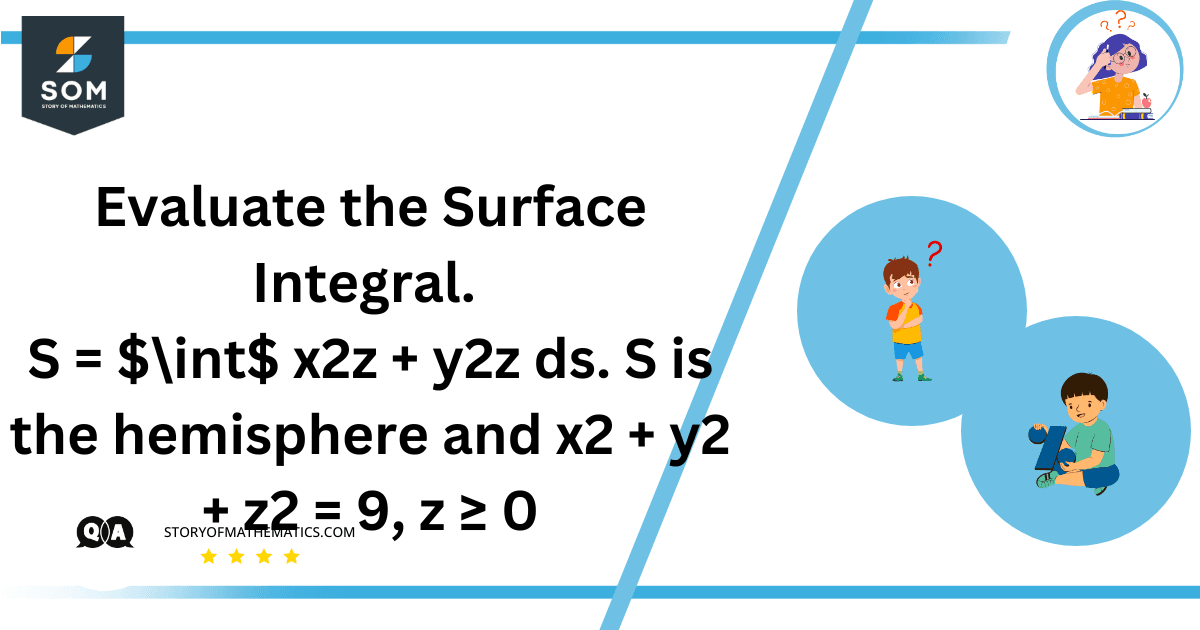# Evaluate the Surface Integral. S = $\int$ x2z + y2z ds. S is the hemisphere and x2 + y2 + z2 = 9, z ≥ 0Calculate the surface integral over a hemisphere defined by $x^2 + y^2 + z^2 = 9$ and z ≥ 0, with the integrand $x^2z + y^2z$. Using spherical coordinates, we parameterize the surface and compute the integral for this geometric region.

To evaluate the surface integral over the hemisphere S defined by $x^2 + y^2 + z^2 = 9$ with z ≥ 0, we can use the parametric representation of the surface. In this case, we can use spherical coordinates to describe the hemisphere:

$x = ρsin(φ)cos(θ)$
$y = ρsin(φ)sin(θ)$
$z = ρcos(φ)$

Here, ρ is the radius of the sphere, which is 3 in this case, and θ and φ are the spherical coordinates.

The surface element dS in spherical coordinates is given by:

$dS = ρ^2sin(φ)dφdθ$

Now, let’s rewrite the integrand in terms of spherical coordinates:

$x^2z + y^2z = (ρsin(φ)cos(θ))^2(ρcos(φ)) + (ρsin(φ)sin(θ))^2(ρcos(φ))$

$= ρ^3sin(φ)cos^2(θ)cos(φ) + ρ^3sin(φ)sin^2(θ)cos(φ)$

Now, we can set up the integral using these expressions:

$\iint S (x^2z + y^2z) dS$

$= \int_{0}^{2\pi} \int_{0}^{\frac{\pi}{2}} [ρ^3sin(φ)cos^2(θ)cos(φ) + ρ^3sin(φ)sin^2(θ)cos(φ)] ρ^2sin(φ) dφ dθ$

$= \int_{0}^{2\pi} \int_{0}^{\frac{\pi}{2}} ρ^5sin(φ)cos(φ)[cos^2(θ) + sin^2(θ)] dφ dθ$

$= ρ^5 \int_{0}^{2\pi} \int_{0}^{\frac{\pi}{2}} sin(φ)cos(φ) dφ dθ$

Now, you can integrate with respect to φ and θ:

$\int_{0}^{2\pi} dθ = 2π$

$\int_{0}^{\frac{\pi}{2}} sin(φ)cos(φ) dφ$

$= \frac{1}{2} \left[ sin^2(φ) \right]_{0}^{\frac{\pi}{2}} = \frac{1}{2}$

So, the surface integral becomes:

$\iint S (x^2z + y^2z) dS$

$= ρ^5 * 2π * 1/2 = 3^5 * 2π * 1/2 = 243π$

Therefore, the value of the surface integral is 243π.

## Example

Evaluate the surface integral. $\iint S (x^2z + y^2z) dS$. S is the hemisphere $x^2 +y^2 + z^2 = 4, z ≥ 0$.

## Solution

$ρ = 2$
$0 ≤ φ ≤ π/2$
$0 ≤ θ ≤ 2π$

Where ρ is the radius of the hemisphere, φ is the polar angle (ranging from 0 to π/2), and θ is the azimuthal angle (ranging from 0 to 2π).

Now, express x, y, and z in terms of spherical coordinates:

$x = ρsin(φ)cos(θ)$
$y = ρsin(φ)sin(θ)$
$z = ρcos(φ)$

The surface element dS in spherical coordinates is given by:

$dS = ρ^2sin(φ)dφdθ$

Now, rewrite the integrand (x^2z + y^2z) in terms of spherical coordinates:

$x^2z + y^2z = (ρsin(φ)cos(θ))^2(ρcos(φ)) + (ρsin(φ)sin(θ))^2(ρcos(φ))$

$= ρ^3sin(φ)cos^2(θ)cos(φ) + ρ^3sin(φ)sin^2(θ)cos(φ)$

Now, set up the integral using these expressions:

$\iint S (x^2z + y^2z) dS$

$= \int_{0}^{2\pi} \int_{0}^{\frac{\pi}{2}} [ρ^3sin(φ)cos^2(θ)cos(φ) + ρ^3sin(φ)sin^2(θ)cos(φ)] ρ^2sin(φ) dφ dθ$

$= \int_{0}^{2\pi} \int_{0}^{\frac{\pi}{2}} ρ^5sin(φ)cos(φ)[cos^2(θ) + sin^2(θ)] dφ dθ$

$= ρ^5 \int_{0}^{2\pi} \int_{0}^{\frac{\pi}{2}} ρ^5sin(φ)cos(φ) dφ dθ$

Now, integrate with respect to φ and θ:

$\int_{0}^{2\pi} dθ = 2π$

$\int_{0}^{\frac{\pi}{2}} sin(φ)cos(φ) dφ$

$= \frac{1}{2} \left[ sin^2(φ) \right]_{0}^{\frac{\pi}{2}} = \frac{1}{2}$

So, the surface integral becomes:

$\iint S (x^2z + y^2z) dS$

$= ρ^5 * 2π * 1/2 = 2^5 * π * 1/2 = 16π$